# How to Forecast Sales Using Historical Data in Excel (6 Methods)

Get FREE Advanced Excel Exercises with Solutions!

Users from all classes somewhere down the line are required to find the forecast data (i.e., Sales, Demand, or anything) depending on available historical data. As a result, users try to forecast sales using historical data in Excel. Excel offers multiple features and functions to forecast sales while maintaining the trend of given data.

Let’s say we have the Sales Amount for the first couple of dates in a month. Now, we want to forecast the sales amount for the rest of the days within the month.In this article, we demonstrate multiple features, FORECAST, TREND, and GROWTH functions as well as the Manual Method to forecast sales using historical data in Excel.

## What Is Forecasting?

Forecasting is a method to generate a correlation between amount/quantity and trends depending on the available historical data. Forecasting is used to understand market trends and able to take rational steps to accommodate the budget or requirements.

⧭ Forecasting is just a way to assume future happenings, not an exact prediction or anything of its kind.

## 6 Easy Ways to Forecast Sales Using Historical Data in Excel

There are several methods to find forecast data in Excel. Each method has its own shortcomings. Follow the below section to clarify your stance and use any of the methods to find necessary forecast data.

### Method 1: Using Forecast Sheet Feature to Forecast Sales Following Historical Data Trend

Excel provides the Forecast Sheet feature in its Data tab. Users can use this feature to find forecast data while maintaining the historical data trend.

Step 1: Highlight the available historical data then go to the Data tab > Click on Forecast Sheet (in the Forecast section).Step 2: Excel fetched the Create Forecast Worksheet window. In the window provide necessary entries in the dialog boxes as depicted. Follow This Link to learn details about those options. Click on Create.🔺 In a moment, Excel inserts a new Worksheet with forecasted Lower and Upper Bound values as shown in the below screenshot.🔺 Also, Excel inserts a Trend Chart depicting the forecasted Lower and Upper Bound along with the Forecast values mimicking the historical data.### Method 2: Using Moving Average to Forecast Sales Considering Historical Data

Moving Average property is found in multiple features of Excel. A typical moving average value is the average value of a dynamic handful (here it is 7) of entries, the Moving Average tool in the Data Analysis feature or the Trendline options’ Moving Average can forecast sales or anything using historical data.

🔄 Typical Moving Average Forecast

Step 1: Use the AVERAGE function to find the average value of 1st 7 entries (i.e., C5:C11) for the 1st forecast value (i.e., 18-May).

`=AVERAGE(C5:C11)`

###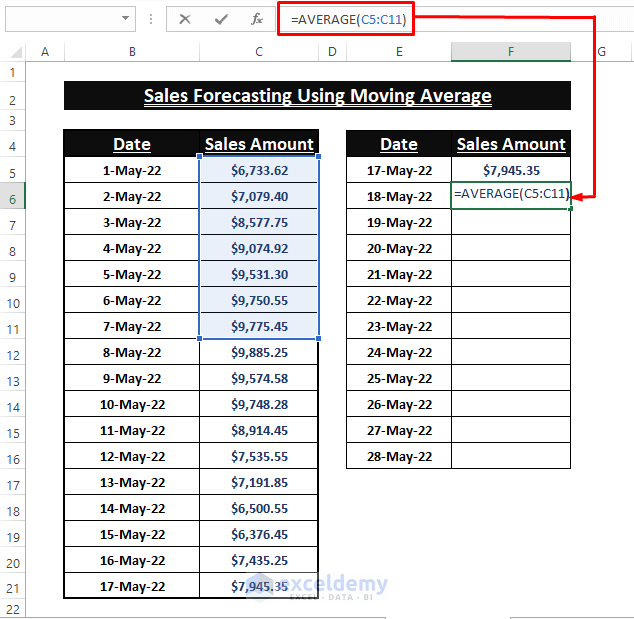Step 2: Press ENTER then use the Fill Handle to display the forecasted values for the rest of the month.

###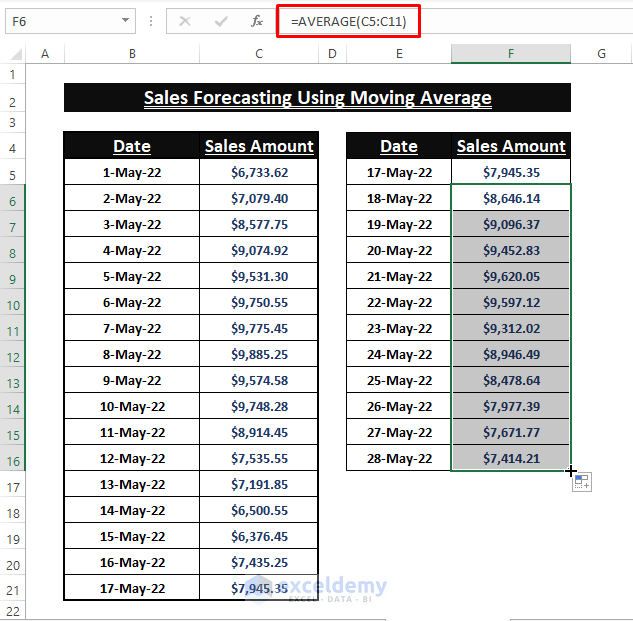🔄 Data Analysis- Moving Average

🔼 Go to the Data tab > Click on the Data Analysis feature in the Data Analysis section. Find and choose the Moving Average option within the Data Analysis tools. Follow This Link for a detailed description of the whole process.

###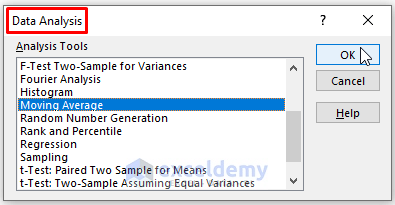🔄 Trendline Options

Users can insert a Moving Average Trendline in the Line Chart depiction of the historical data. To do so, users 1st have to insert a Line Chart of the available historical data.

Step 1: Move to Insert > Select Line (from the Charts section). Excel automatically takes the existing data and inserts a Line Chart.

###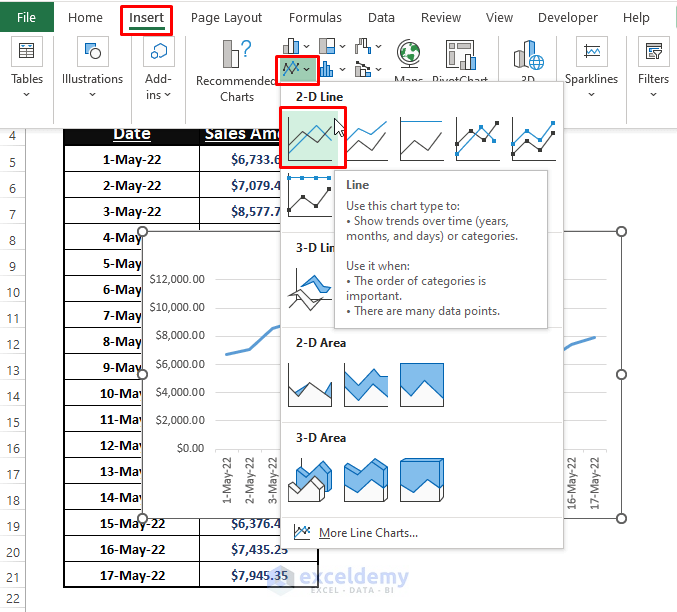Step 2: Click on the Plus icon beside the Chart > Tick the Trendline option and click on the Arrow > Select More Options.Step 3: The Format Trendline window appears. Click on the Moving Average from the Format Trendline Options.

###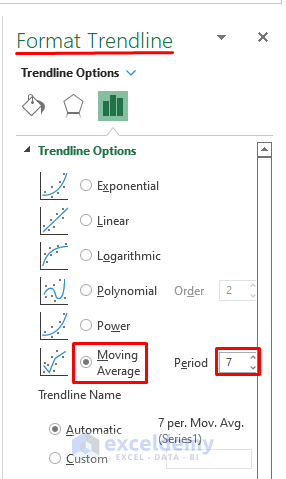🔺 Furnish the Line Chart as you desire. In the end, the forecasted values will be depicted as a Line (here it’s the red line) in the Chart.### Method 3: Using FORECAST Function Variants to Forecast Sales

There are multiple variants of the FORECAST function. Plain FORECAST, FORECAST.LINEAR, FORECAST.ETS are some of the FORECAST function’s variants. These functions are used to forecast sales using historical data in Excel.

🔄FORECAST Function

The FORECAST function returns the linear trends outcomes maintaining the historical data. The syntax of the function is

`FORECAST (x, known_ys, kown_xs)`

🔼 Paste the below formula in the desired cells to find forecasted data.

`=FORECAST(E5:E18,C5:C21,B5:B21)`🔼 After the formula insertion press CTRL+SHIFT+ENTER to display the forecasted values.🔄FORECAST.LINEAR Function

Alternative to the FORECAST function, the FORECAST.LINEAR function returns the future values mimicking the historical data trend. The syntax of the function is

`FORECAST.LINEAR (x, known_ys, kown_xs)`

🔼 Use the below formula in any cell then hit CTRL+SHIFT+ENTER. You get the forecasted data instantly.

`=FORECAST.LINEAR(E5:E18,C5:C21,B5:B21)`🔄FORECAST.ETS Function

For allowing the seasonality trends in forecasted data, it’s preferable to use the FORCAST.ETS function. The syntax of the FORECAST.ETS function is

`FORECAST.ETS (target_date, values, timeline, [seasonality], [data_completion], [aggregation])`

🔼 Type the following formula in any cell then use the CTRL+SHIFT+ENTER key to apply the formula. The formula returns the forecasted data considering the seasonality in historical data trends.

`=FORECAST.ETS(E5:E18,C5:C21,B5:B21)`🔼 Alternative to the FORECAST.ETS function, there is an Exponential Smoothing tool in the Data Analysis feature. Go through This Link to learn the details regarding its application.### Method 4: Forecast Sales Using Historical Data Using TREND Function

The TREND function fits the forecast data to the linear trend of the historical data. The function’s outcomes are the linear trend values that sit on a straight line. The syntax of the function is

`TREND(known_y's, [known_x's], [new_x's], [const])`

🔼 Type the following formula in any cell.

`=TREND(C5:C21,B5:B21,E5:E18)`🔼 Press CTRL+SHIFT+ENTER altogether to display the outcomes.### Method 5: Forecast Sales Using GROWTH Function

Exponential growth is predicted by the GROWTH function. The function delivers outcomes that follow exponential growth using historical data. The syntax of the function is

`GROWTH(known_y's, [known_x's], [new_x's], [const])`

🔼 Write the following formula in any desired cell.

`=GROWTH(C5:C21,B5:B21,E5:E18)`🔼 As the function is an array function, use CTRL+SHIFT+ENTER to display the outcomes.Use ENTER in case of array formulas if you are using the Excel 365 version.

### Method 6: Using Manual Method to Forecast Sales Maintaining Historical Data Trend

Suppose we have 1st six months sales amount as period data. We want to use these sales data to forecast the next six months’ sales.Step 1: Use the below LINEST array formula to find the constant values of a linear equation. If the linear equation is Y= bX+c, the function results in both constant b and c.

`=LINEST(C8:C13,D8:D13,TRUE,FALSE)`Step 2: Now, find the trend values of monthly sales. Paste the below formula in cell E8.

`=\$C\$5*D8+\$D\$5`Step 3: Use the Fill Handle to populate the trend values. Afterward, find the deviation of the actual value from the trend. Divide Actual Value by the Trend one.

`=C8/E8`Step 4: After that calculate the Average Sales (using the AVERAGE function) and Seasonality Index (Sales Amount/Average Sales) and Average Seasonality Index (using the AVERAGE function).Step 5: Next, find the trend value for the next 6 months using the below formula.

`=\$T\$5*T8+\$U\$5`Step 6: Finally, multiply the seasonality index with trend values to find the forecast sales amount taking seasonality into account.

`=\$S\$8*U8`You can use a huge historical dataset to allow Excel to mimic more efficiently. As we have representation limitations, we use a comparatively smaller historical dataset.

## Conclusion

In this article, we demonstrate multiple features and functions to forecast sales using historical data in Excel. It’s hard to justify the forecast values without putting them to test with real data. These above-mentioned methods have their limitations as some of them take into account the seasonality some don’t. Hope this article clarifies users’ understanding of forecasting and sheds some light to find desired directions. Comment, if further inquiries arose or have anything to say.

## Related Articles#### Maruf Islam

My self, Maruf Islam, an engineer and Excel & VBA Content developer on Exceldemy. I enjoy solving problems, finding workable solutions, and most of the part I really like to take on challenges. On Exceldemy I write articles discussing various way outs of Microsoft Excel's stuck ons.

We will be happy to hear your thoughtsAdvanced Excel Exercises with Solutions PDF